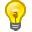PHP变量是什么？

1、在PHP中变量是用于储存信息的容器，类似于数学中的集合。

2、赋值方法与数学中的代数相类似。

```<?php
\$x=5;
\$y=6;
\$z=\$x+\$y;
echo \$z;
?>```

## 与代数类似

x=5
y=6
z=x+y变量是用于存储数据的容器。

## PHP 变量

PHP 变量规则：

• 变量以 \$ 符号开始，后面跟着变量的名称

• 变量名必须以字母或者下划线字符开始

• 变量名只能包含字母数字字符以及下划线（A-z、0-9 和 _ ）

• 变量名不能包含空格

• 变量名是区分大小写的（\$y 和 \$Y 是两个不同的变量）PHP 语句和 PHP 变量都是区分大小写的。

PHP 没有声明变量的命令。

## 实例

```<?php
\$txt="Hello world!";
\$x=5;
\$y=10.5;
echo \$txt;
?>```

## PHP 是一门弱类型语言

PHP 会根据变量的值，自动把变量转换为正确的数据类型。

PHP 有四种不同的变量作用域：

• local

• global

• static

• parameter

## 实例

```<?php
\$x=5; // 全局变量

function myTest()
{
\$y=10; // 局部变量
echo "<p>测试函数内变量:<p>";
echo "变量 x 为: \$x";
echo "<br>";
echo "变量 y 为: \$y";
}

myTest();

echo "<p>测试函数外变量:<p>";
echo "变量 x 为: \$x";
echo "<br>";
echo "变量 y 为: \$y";
?>```你可以在不同函数中使用相同的变量名称，因为这些函数内定义的变量名是局部变量，只作用于该函数内。

## PHP  global 关键字

global 关键字用于函数内访问全局变量。

## 实例

```<?php
\$x=5;
\$y=10;

function myTest()
{
global \$x,\$y;
\$y=\$x+\$y;
}

myTest();
echo \$y;
?>```

PHP 将所有全局变量存储在一个名为 \$GLOBALS[index] 的数组中。 index 保存变量的名称。这个数组可以在函数内部访问，也可以直接用来更新全局变量。

## 实例

```<?php
\$x=5;
\$y=10;

function myTest()
{
\$GLOBALS['y']=\$GLOBALS['x']+\$GLOBALS['y'];
}

myTest();
echo \$y;
?>```

## 实例

```<?php

function myTest()
{
static \$x=0;
echo \$x;
\$x++;
}

myTest();
myTest();
myTest();

?>```

## 实例

```<?php

function myTest(\$x)
{
echo \$x;
}

myTest(5);

?>```